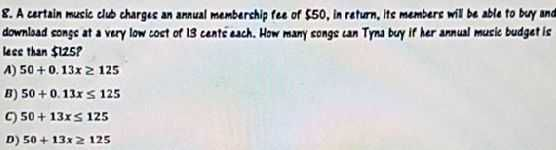### ¿Todavía tienes preguntas de matemáticas?

Pregunte a nuestros tutores expertos
Algebra
PreguntaA certain music club charges an annual membership fee of $$\ 50$$ , in return, its members will be able to buy and download songs at a very low cost of $$13$$ cents each. How many songs can Trma buy if her annual music budget is less than $$\ 125$$ ?

A) $$50 + 0.13 x \geq 125$$

B) $$50 + 0.13 x \leq 125$$

C) $$50 + 13 x \leq 125$$

D) $$50 + 13 x \geq 125$$

B) $$50+ 0.13x\le 125$$